# How many daL of bromine atoms are in 90 x 1022 bromine atoms? (The density of bromine is 3.1 g/cm3)

How many daL of bromine atoms are in 90 x 1022 bromine atoms?  (The density of bromine is 3.1 g/cm3)

We need at least 10 more requests to produce the answer.

0 / 10 have requested this problem solution

The more requests, the faster the answer.

All students who have requested the answer will be notified once they are available.

#### Earn Coin

Coins can be redeemed for fabulous gifts.

Similar Homework Help Questions
• ### How many daL of bromine are in 15.7 x 1022 molecules? (The density of bromine is 3.1 g/cm3)

How many daL of bromine are in 15.7 x 1022 molecules?  (The density of bromine is 3.1 g/cm3)

• ### 41. Suppose you have half a mole of sulfur atoms. How many sulfur atoms do you...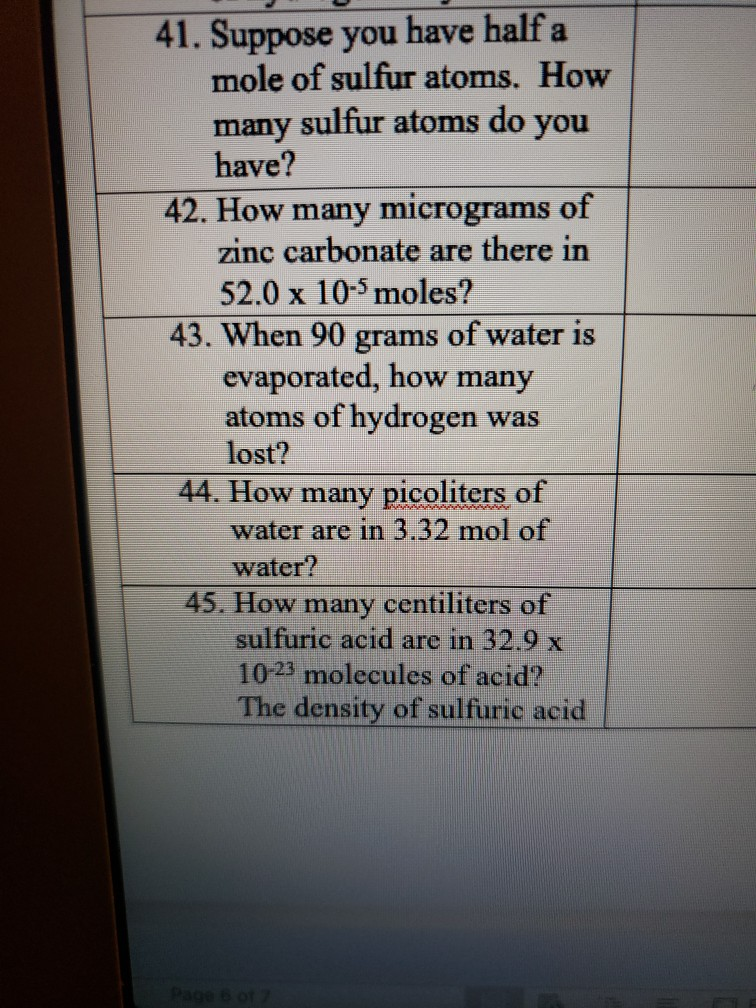41. Suppose you have half a mole of sulfur atoms. How many sulfur atoms do you have? 42. How many micrograms of zinc carbonate are there in 52.0 x 10-5 moles? 43. When 90 grams of water is evaporated, how many atoms of hydrogen was lost? 44. How many picoliters of water are in 3.32 mol of water? 45. How many centiliters of sulfuric acid are in 32.9 x 10-23 molecules of acid? The density of sulfuric acid Page 6...

• ### The density of solid FeFe is 7.87 g/cm3.7.87 g/cm3. How many atoms are present per cubic...

The density of solid FeFe is 7.87 g/cm3.7.87 g/cm3. How many atoms are present per cubic centimeter (cm3)(cm3) of Fe?Fe? FeFe atoms: atoms/cm3atoms/cm3 As a solid, FeFe adopts a body‑centered cubic unit cell. How many unit cells are present per cubic centimeter (cm3)(cm3) of Fe?Fe? unit cells: unit cells/cm3unit cells/cm3 What is the volume of a unit cell of this metal? volume: cm3cm3 What is the edge length of a unit cell of Fe?Fe? edge length: cm

• ### How many bromine atoms are present in 26.0 g of CH2Br2 ??

How many bromine atoms are present in 26.0 g of CH2Br2 ??

• ### How many moles are each of the following? (a) 3.17 x 1022 atoms of C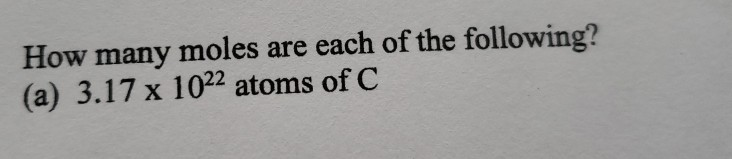How many moles are each of the following? (a) 3.17 x 1022 atoms of C

• ### The density of iron is 7.86 grams/cm3 . How many iron atoms would be found in...

The density of iron is 7.86 grams/cm3 . How many iron atoms would be found in 1.00 cubic nanometer (1.00 nm3 )? (1 m = 109 nm; 1 m = 100 cm).

• ### 4. How many moles of hydrogen atoms are needed to make in 4.45 x 1022 formula...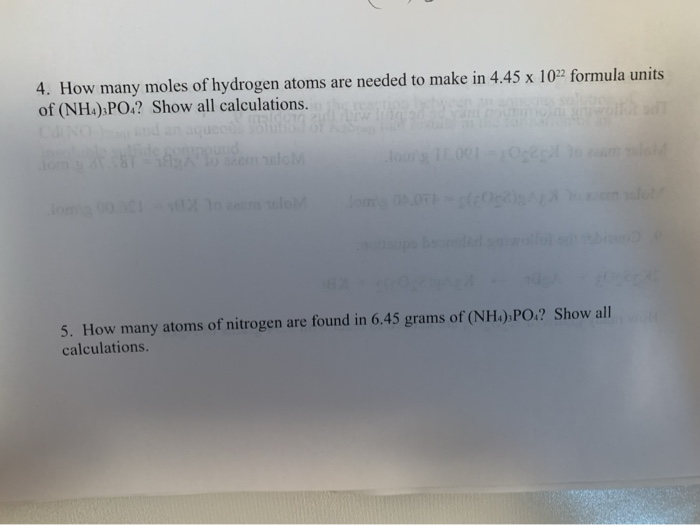4. How many moles of hydrogen atoms are needed to make in 4.45 x 1022 formula units of (NHa) PO.? Show all calculations. CANO ulob o 0.07 5. How many atoms of nitrogen are found in 6.45 grams of (NH4), PO,? Show all calculations

• ### metallic iron crystallizes in a cubic lattice, the unit cell edge length is 287pm, the density of iron is 7.87 g/cm3, how many iron atoms are there within a unit cell

metallic iron crystallizes in a cubic lattice, the unit cell edge length is 287pm, the density of iron is 7.87 g/cm3, how many iron atoms are there within a unit cell? a few steps of how it is worked out would be ok

• ### 51. How many atoms of aluminum can be produced by the decomposition of 13.3 g of...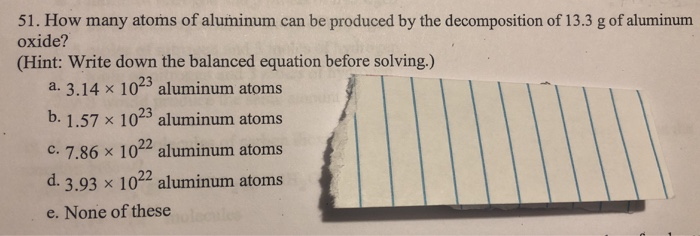51. How many atoms of aluminum can be produced by the decomposition of 13.3 g of aluminum oxide? (Hint: Write down the balanced equation before solving.) a. 3.14 x 1023 aluminum atoms b. 1.57 % 1023 aluminum atoms c. 7.86 x 1022 aluminum atoms d. 3.93 x 1022 aluminum atoms e. None of these

• ### How many hydrogen atoms are in 135 g of H20? Multiple Choice o 170 atoms o...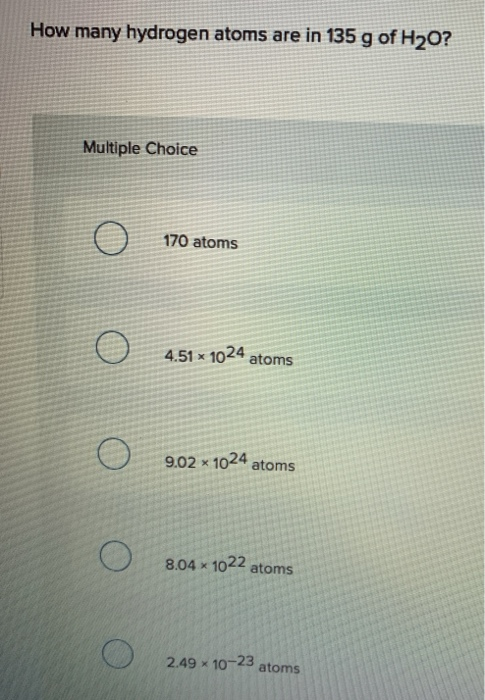How many hydrogen atoms are in 135 g of H20? Multiple Choice o 170 atoms o 4.51 x 1024 atoms o 9.02 x 1024 atoms o 8.04 x 1022 atoms o 2.49 х 10-23 atoms